# Latin rectangle

(diff) ← Older revision | Latest revision (diff) | Newer revision → (diff)

A rectangular matrix of dimension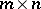,, each row of which is a permutation (without repetitions) of the elements of a setconsisting ofelements, and in the columns each element occurs at most once. For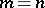a Latin rectangle is a Latin square of order. Usually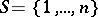, and one says that the Latin rectangle is constructed on the set.

A Latin rectangle exists for any natural numbersand,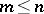. An example of a Latin rectangle is a matrix with first row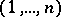and where any subsequent row is obtained from the previous row by a cyclic shift by one place. A Latin rectangle of dimension,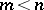, can always be completed to a Latin square of orderin such a way that the firstrows of the Latin square are the same as those of the Latin rectangle.

For the numberof Latin rectangles of dimensionone has the following lower bound: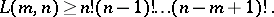A Latin rectangle is said to be normalized if its first row is. The numberof normalized Latin rectangles is connected with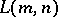by the relation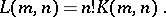The calculation offor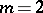and 3 is connected with the following classical combinatorial problems: the problem of the number of derangements (see also Inversion (in combinatorics)) and the married-couples problem. Thus, the number of derangements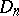equals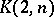, and the number of arrangements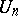in the married-couples problem is the number of Latin rectangles of dimensionwith first two rows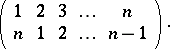Forone has the formulas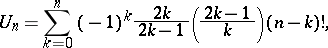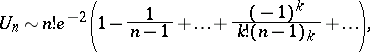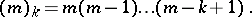The numbercan be expressed in terms ofand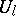:where,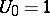. One also has the following asymptotic expansion:where,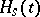being the Hermite polynomials. It is also known that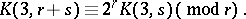The problem of enumerating Latin rectangles having more than three rows is unsolved (1982). For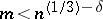, wheresuch that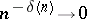, the following asymptotic behaviour holds: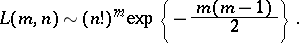Certain concepts and theorems connected with Latin squares can be extended to Latin rectangles. Thus, two Latin rectangles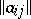and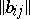of dimensionare said to be orthogonal if all pairs of the form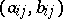are distinct. A set of Latin rectangles in which any two of them are orthogonal has at most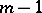Latin rectangles.

The term "Latin rectangle" is often used in a more general sense: A generalized Latin rectangle of dimension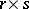, constructed on a setconsisting ofelements, is a matrix of dimensionwith elements fromthat occur at most once in each row and column. A (generalized) Latin rectangle of dimensionconstructed onsymbols can be extended to a Latin square of orderif and only if each symbol occurs at least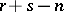times in the Latin rectangle.